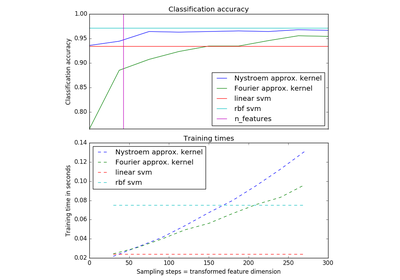# `sklearn.kernel_approximation`.RBFSampler¶

class `sklearn.kernel_approximation.``RBFSampler`(gamma=1.0, n_components=100, random_state=None)[source]

Approximates feature map of an RBF kernel by Monte Carlo approximation of its Fourier transform.

It implements a variant of Random Kitchen Sinks.

Read more in the User Guide.

Parameters: gamma : float Parameter of RBF kernel: exp(-gamma * x^2) n_components : int Number of Monte Carlo samples per original feature. Equals the dimensionality of the computed feature space. random_state : {int, RandomState}, optional If int, random_state is the seed used by the random number generator; if RandomState instance, random_state is the random number generator.

Notes

See “Random Features for Large-Scale Kernel Machines” by A. Rahimi and Benjamin Recht.

 “Weighted Sums of Random Kitchen Sinks: Replacing minimization with randomization in learning” by A. Rahimi and Benjamin Recht. (http://people.eecs.berkeley.edu/~brecht/papers/08.rah.rec.nips.pdf)

Methods

 `fit`(X[, y]) Fit the model with X. `fit_transform`(X[, y]) Fit to data, then transform it. `get_params`([deep]) Get parameters for this estimator. `set_params`(\*\*params) Set the parameters of this estimator. `transform`(X[, y]) Apply the approximate feature map to X.
`__init__`(gamma=1.0, n_components=100, random_state=None)[source]
`fit`(X, y=None)[source]

Fit the model with X.

Samples random projection according to n_features.

Parameters: X : {array-like, sparse matrix}, shape (n_samples, n_features) Training data, where n_samples in the number of samples and n_features is the number of features. self : object Returns the transformer.
`fit_transform`(X, y=None, **fit_params)[source]

Fit to data, then transform it.

Fits transformer to X and y with optional parameters fit_params and returns a transformed version of X.

Parameters: X : numpy array of shape [n_samples, n_features] Training set. y : numpy array of shape [n_samples] Target values. X_new : numpy array of shape [n_samples, n_features_new] Transformed array.
`get_params`(deep=True)[source]

Get parameters for this estimator.

Parameters: deep : boolean, optional If True, will return the parameters for this estimator and contained subobjects that are estimators. params : mapping of string to any Parameter names mapped to their values.
`set_params`(**params)[source]

Set the parameters of this estimator.

The method works on simple estimators as well as on nested objects (such as pipelines). The latter have parameters of the form `<component>__<parameter>` so that it’s possible to update each component of a nested object.

Returns: self :
`transform`(X, y=None)[source]

Apply the approximate feature map to X.

Parameters: X : {array-like, sparse matrix}, shape (n_samples, n_features) New data, where n_samples in the number of samples and n_features is the number of features. X_new : array-like, shape (n_samples, n_components)

## Examples using `sklearn.kernel_approximation.RBFSampler`¶Explicit feature map approximation for RBF kernels